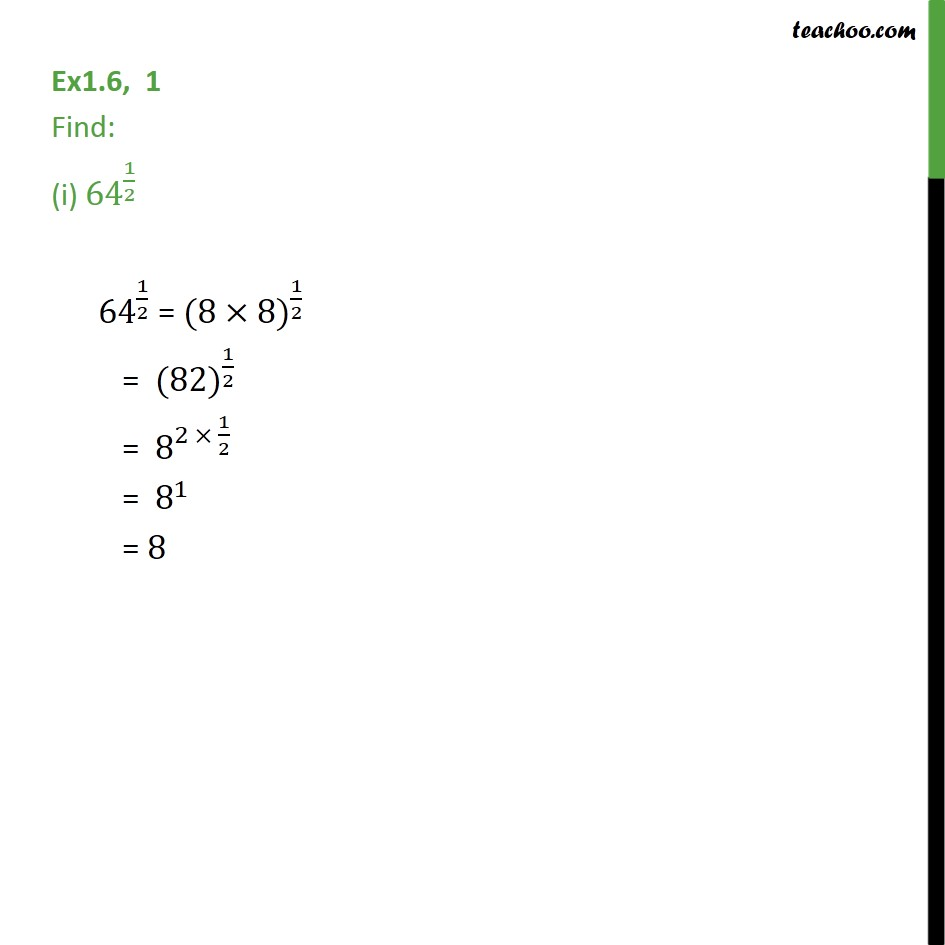1. Chapter 1 Class 9 Number Systems (Term 1)
2. Concept wise
3. Laws of exponents

Transcript

Ex1.6, 1 Find: (i) 〖64〗^(1/2) 〖 64〗^(1/2) = 〖(8×8)〗^(1/2) = 〖 (82)〗^(1/2) = 〖 8〗^(2 × 1/2) = 〖 8〗^1 = 8